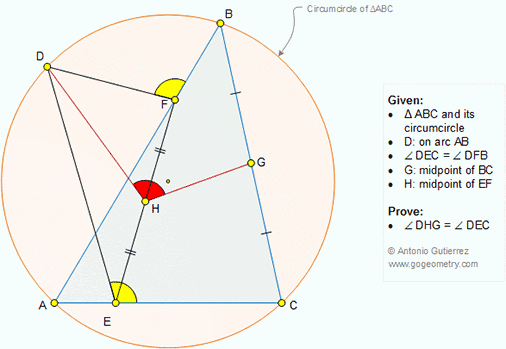Wednesday, July 4, 2018

Geometry Problem 1359: Triangle, Circumcircle, Congruent Angles, Midpoints

Geometry Problem. Post your solution in the comment box below.
Level: Mathematics Education, High School, Honors Geometry, College.

Details: Click on the figure below.1.https://photos.app.goo.gl/v33PQpQuJdPbRY1aA

Connect DA, DC, DB
Let angle(DBA)=angle(DCA)= u
Note that triangle (DFB) similar to triangle(DEC) … ( case AA)
So DE/DF= DC/DB and angle(EDC)=angle(FDB) , angle (EDF)= angle (CDB)
So triangle(EDF) similar to \$(CDB) …(case AA)
And \$(CDB) is the image of \$(EDF) in the spiral similarity transformation with center D, angle od rotation = angle (EDC)
And dilation factor= DC/DE
Median DG is the image of median DH in this transformation so \$(DEC) similar to \$(DHG)
So angle (DHG)= angle (DEC)

2.Considering usual triangle notations, Join DC and DB
Since m(AED)=m(AFD) => ADFE are concyclic
=>m(EDF)=m(EAF)=m(CAB)=m(CDB)=m(A)
Also m(DEF)=m(DAF)=m(DAB)=m(DCB)
Therefore triangles DEF and DCB are similar
and m(EFD)=m(CBD) -----------(1)

Connect DH and DG, which form the medians of the two similar triangles DEF and DCB
=> DH/FE=DG/BC
=> DH/FH=DG/BG --------(2)
From (2) and (1) triangles DHF and DGB are similar
=> DF/DH=DB/DG
=> DF/DB=DH/DG -----------(3)
From (3) triangles DFB and DHG are similar

Hence m(DHG)=m(DFB)=m(DEC)